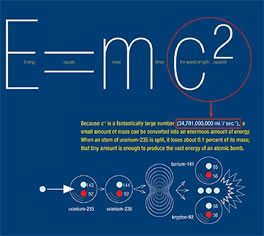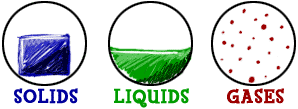# Difference Between Mass and Matter

##### Key Difference: Mass is divided into three types: inertial mass, active gravitational mass and passive gravitational force. The most common type used in physics is the inertial mass, which is a quantitative measure of an object's resistance to acceleration. In the scientific world, matter is defined any object that has mass or volume (occupies space).

Mass and matter are important principles that are most commonly heard in the fields of physics, cosmology and astrophysics. Due to their close relation to each other, they are often assumed to be interchangeable, but they are complete different words with different meanings. Compared to matter, mass is a more clearly defined concept.Mass is divided into three types: inertial mass, active gravitational mass and passive gravitational force. The most common type used in physics is the inertial mass, which is a quantitative measure of an object's resistance to acceleration. Active gravitational mass is a measure of magnitude of the gravitational force which is exerted by an object, while passive gravitational force is a measure of magnitude of the gravitational force which is experienced by an object when interactive with another object. The International System of Units (SI) unit used to denote mass is the kilogram (kg). While the Imperial units system uses pounds, grain and stone to denote mass.

In everyday usage, we use the term “mass” as “weight” which is more closely related to matter than mass. Weight is actually measured in newtowns and not kg. Weight is actually the gravitational force that is acting on a body, while mass is the intrinsic property that never changes. In layman terms, the weight of an object can vary according to the surrounding, while the mass never changes. For example, on Earth a person has a mass of 50 kg and a weight of 491 newtons. The same person on the Moon will have the same mass, but will only weight 81.5 newtons.

Matter and energy are two forms of mass. According to Einstein’s Theory of Relativity, electromagnetic waves also have mass. There are two types of mass: rest mass and relativistic mass. According to the theory, mass of an object does not always remain constant; the rest mass is the mass of an object is at rest, while a relativistic mass when the object is in motion. Mass can also be converted into energy which is used in nuclear power generation.Though, matter does not have a proper scientific definition, the concept of matter goes back to the Ancient Greeks. In those days, matter was considered as ‘material’, meaning everything that was tangible was considered as matter. In the scientific world, matter is defined any object that has mass or volume (occupies space). The oldest real scientific theory of matter is believed to be suggested by Leucippus and Democritus in around early 400 BC. The “particulate theory of matter” theory states that matter is not continuous, but built of discrete building blocks.

Matter is usually classified in four states or phases: solid, liquid, gas and plasma. It was stated that all objects are made up of molecules and each molecule is made up of atom, atom matter, made up of interacting subatomic particles. However, Einstein’s theory of relativity stated that all matter can eventually turn into energy. He showed that waves sometimes behaved as particles and particles behaved as waves. This is known as the wave particle duality. This combined mass and energy, making them forms of matter. The equation E=mc2, where E is the energy of a piece of matter of mass m, times c2 the speed of light squared, shows much energy can be obtained from a piece of matter.

When matter is heated enough it ionizes (or loses its electrons), causing it to emit energy in the form of light. The light that is given off by the sun and starts is a result of this ionization. Atomic matter at lower temperatures also can reflect light, absorbing some at specific wavelengths, which determines the colors of the objects we see.